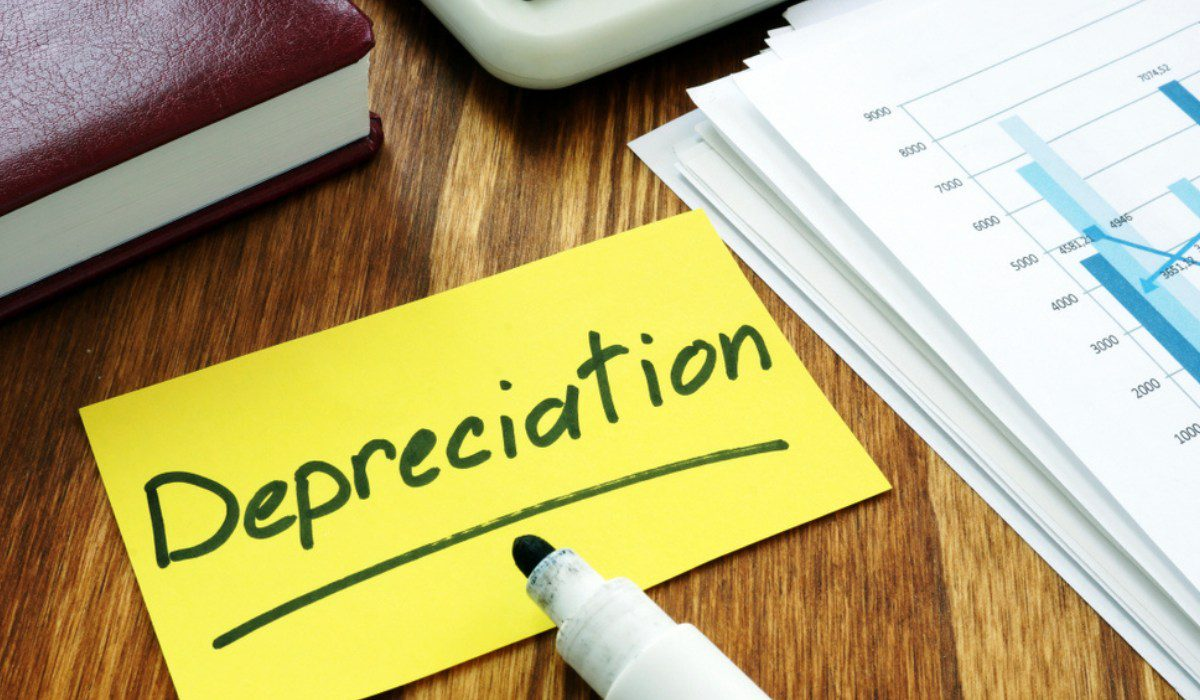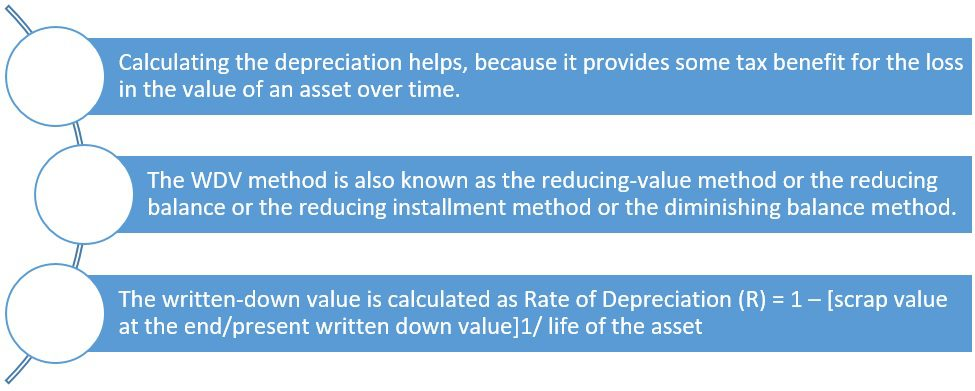What does ‘written-down value’ of an asset mean?The written-down value is one of the methods used to calculate the depreciation in the value of an asset, for income tax purposes

To calculate the depreciation of an asset, experts turn to two methods of valuation – the Straight Line Method (SLM) and the Written Down Value (WDV) method. Of these, the WDV method is used for income tax purposes.

What is the WDV method?

After accounting for its depreciation or amortisation, accountants arrive at the WDV of an asset. In short, it is the current value of the asset.Why is depreciation calculated?

Due to wear and tear of an asset, there might be a loss in the value of the asset over time. Section 32 of the Income Tax Act of 1961, deals with such depreciation in the value of an asset. Depreciation is calculated for tax purposes and the Act allows calculation for both, tangible (such as building, factory plant, machinery) and intangible assets (trademarks, patents, franchise).

So how does calculating depreciation help? Know that if an asset is used for over 180 days, a 50% depreciation is allowed for the year. It is not required that the asset has to be mandatorily used in the previous year. If the asset was leased to the lessee, the assessee can claim deduction under the I-T Act.

Calculating the depreciation helps, because it provides you some tax benefit. Companies are also required to calculate it, to ascertain profits and losses. In the absence of such calculations, companies may not have any indicator of real profit and may suffer due to wrong valuations.

See also: What is a real estate appraisal and how does it work?

Rate of depreciation of common assets

Building for residential use: 5%

Building for non-residential use: 10%

Furniture and fittings: 10%

Computers including software: 40%

Plant and machinery: 15%

Motor vehicles for personal use: 15%

Motor vehicles for commercial use: 30%

All intangible assets: 25%.

Other terms used in place of written-down value

The WDV method is also known as the reducing-value method or the reducing balance or the reducing installment method or the diminishing balance method.

Formula to calculate depreciation through WDV method

The WDV method is considered the most logical method. An asset is considered to provide more value in the initial years than the later years.

Rate of Depreciation (R) = 1 – [s/c]1/n

Where, ‘s’ stands for the scrap value at the end of the period, that is ‘n’.

‘c’ stands for the written down value at present.

‘n’ is the useful life of the asset.

Note: The useful life of an asset, for different asset classes is provided in the Schedule II of Companies Act. Useful life of buildings (other than factory buildings) with RCC frame structure is 60 years and that of buildings (other than factory buildings) other than RCC frame structure is 30 years.

In the WDV method, depreciation is charged on the book value of such an asset and every year, the book value decreases. Let us see this through an example:

Suppose the cost of the asset is Rs 1,00,000.

Depreciation for the first year – 10%

So, depreciation is Rs 10,000 for the first year.

Depreciation for the second year = Rs 10,000 (10% of Rs 90,000) = Rs 9,000

Depreciation for the third year = 10% of Rs 81,000 [i.e., 90,000 – 9,000] = Rs 8,100

Demerits of opting for the WDV method

Although the WDV method is the most practical and preferred method to calculate depreciation, it has its own limitations. For example, year after year the original cost of the asset escapes attention. Secondly, the asset can never be brought down to zero.

Moreover, any interest on the capital which is invested in the asset is also not taken into account. This method also requires extensive book-keeping and yet, arriving at the correct value may be a difficult task. However, if you have to calculate the depreciation of a plant, machinery or even a vehicle, the WDV method is the best.

FAQs

Which are some of the methods commonly used to calculate depreciation of assets?

Some of the popular methods used to calculate depreciation include the Straight Line Method, Written Down Value Method, Unit of Production Method, Double Declining Balance Method and Sum-of-the-year's Digits method.

When is the WDV method best suited to calculate depreciation?

For fixed assets that go through maximum wear and tear, the WDV method of calculating its depreciation works best.

What does a write down mean?

An accounting term ‘write-down’ refers to the reduction in the book value of an asset.